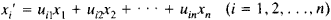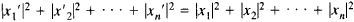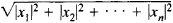# Unitary Transformation

(redirected from Antiunitary transformation)

## unitary transformation

[′yü·nə‚ter·ē ‚tranz·fər′mā·shən]
(mathematics)
A linear transformation on a vector space which preserves inner products and norms; alternatively, a linear operator whose adjoint is equal to its inverse.
McGraw-Hill Dictionary of Scientific & Technical Terms, 6E, Copyright © 2003 by The McGraw-Hill Companies, Inc.
The following article is from The Great Soviet Encyclopedia (1979). It might be outdated or ideologically biased.

## Unitary Transformation

a linear transformationwith complex coefficients that leaves invariant the sum of the squares of the absolute values of the transformed quantitiesSince it preserves the lengthof a vector x with components x1,x2, . . ., xn, a unitary transformation is the extension to a complex n-dimensional vector space of the notion of a rotation in the Euclidean plane or in Euclidean 3-space. The coefficients of a unitary transformation form a unitary matrix. The unitary transformations of an n-dimensional complex space form a group under multiplication. If the coefficients uij and the transformed quantities xi are real, then the unitary transformation reduces to an orthogonal transformation of an n-dimensional real vector space.